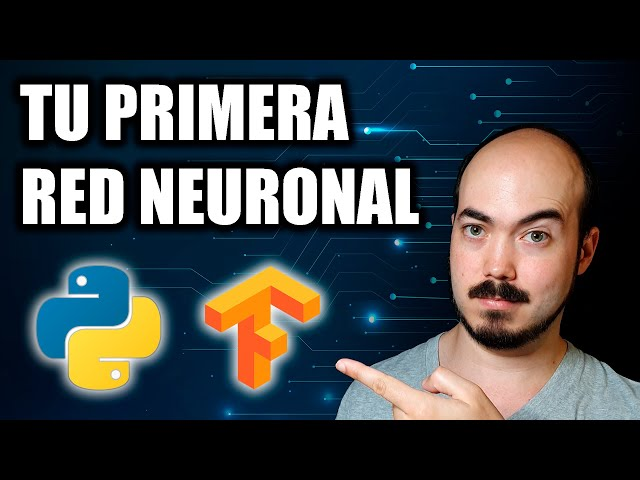# Python and TensorFlow: Create Your First Neural NetworkTLDR The video explains how machine learning and neural networks can be used to create models that can learn on their own to perform tasks, such as converting Celsius to Fahrenheit, and provides a step-by-step guide on how to program in Python for AI using Google Colab and TensorFlow.

## Key insights

• 🧠
Machine learning works conceptually differently from regular programming, allowing us to do complex and amazing things.
• 🧠
Machine learning is useless when we don't know the formula or algorithm, but neural networks can learn the algorithm by themselves with the right data.
• 🧐
The magic of machine learning lies in its ability to learn appropriate values for weights and biases without manual trial and error.
• 💻
The optimizer algorithm "adam" lets the network know how to adjust weights and biases efficiently so that it learns and improves little by little.
• 📈
The function loss can be used to evaluate the performance of a neural network during training and determine when further training is no longer necessary.
• 🤖
The network was able to reach an exact result without being told the formula, showcasing the power of neural networks in making predictions.
• 🤖
Complex neural networks can learn algorithms to solve simple problems in different ways, showcasing the potential of AI in problem-solving.

## Q&A

• What is the difference between machine learning and regular programming?

Machine learning involves creating a model that can learn by itself to convert inputs into results, unlike regular programming where the algorithm is already known.

• How can machine learning be used to convert Celsius to Fahrenheit?

Machine learning can teach a neural network to learn the algorithm for converting Celsius to Fahrenheit without knowing the formula by providing enough examples of inputs and results.

• What is Google Colab and how is it used for artificial intelligence programming?

Google Colab is an online notebook that allows you to program in Python and use CPU and GPU of a computer in the cloud for free without installing anything, and in this lecture, the speaker explains how to use it step by step for artificial intelligence programming.

• What are the important properties in training a model for automatic learning?

The optimizer "adam" adjusts weights and biases efficiently and the learning rate determines how much to adjust, while the loss function "min error" considers a few large errors to be worse than a large number of small errors.

• What is the next step in artificial vision after using complex neural networks?

The next step in artificial vision is using convolution neural networks to detect and classify images.

## Timestamped Summary

• 🧠
00:00
Machine learning is about creating a model that can learn on its own to produce results, unlike regular programming where the algorithm is already known.
• 🧠
01:41
Using machine learning, a neural network can learn to convert Celsius to Fahrenheit without knowing the formula.
• 🧠
03:58
Neural networks can learn to perform tasks like converting Celsius to Fahrenheit by adjusting weights and biases based on examples given through machine learning.
• 👨‍💻
06:27
Use Google Colab for free cloud computing and learn step-by-step how to program in Python for AI.
• 🧮
07:52
Create a TensorFlow neural network model to convert Celsius to Fahrenheit with a dense layer and sequential model.
• 🤖
09:11
The key to training a model for automatic learning is using the "adam" optimizer and "min error" loss function to efficiently adjust weights and biases while minimizing errors.
• 📈
10:23
The model is trained with 1000 laps, but after 500 or 600 laps, it is ready to make predictions.
• 🧠
11:57
The successful training of a neural network using a linear function to predict Celsius to Fahrenheit conversion, with the potential for more complex networks to solve problems in different ways and the use of convolution neural networks for image detection and classification.Report the articleThank you for the feedback